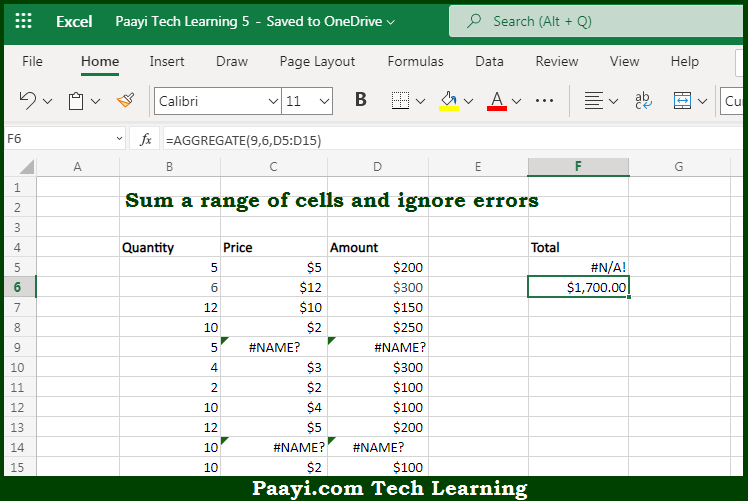# Learn How to SUM and Ignore Errors in Microsoft Excel

Written by | 0 Comments | 561 Views

In this article, you will learn how to COUNT various things in Microsoft Excel using a single/combination(s) of functions. You will also know how to SUM and Ignore Errors and see the generic formula.

SUM and Ignore Errors in Microsoft Excel

The main purpose of this formula is to sum a range of cells and ignore errors. Here we will learn how to sum and ignore errors in Microsoft Excel. That implies, with the help of a formula based on the  AGGREGATE function you can able to sum a range of cells and ignore errors. So, with the help of this formula, you can able to sum and ignore errors in Microsoft Excel.

General Formula to SUM and Ignore Errors

=AGGREGATE(9,6,data)

The Explanation for the SUM and Ignore ErrorsSo we know that with the help of the given formula above you can able to sum a range of cells and ignore errors. Here we will learn how to sum and ignore errors in Microsoft Excel. As we know that the most common problem in Microsoft Excel is that errors in data come up in summary calculations. In fact, the errors can be resolved by entering the missing data, and the SUM function will start working again. But, it is often helpful when summary calculations display errors because it signals there are problems in the data that should be viewed once again. Still, there are situations where you want to ignore errors and sum the available numbers. So, with the help of this formula, you can able to sum a range of cells and ignore errors.